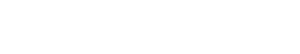# Geometry

Geometry is the study of shapes, sizes, and relative positions of objects in space. In text analytics, geometry may be used to analyze the relative positions of words in a document. For example, geometry can be used to calculate the distance between two words in a document. Geometry can also be used to determine the orientation of words in a document. For example, geometry can be used to calculate the angle between two words in a document.

Geometry is similar to other terms used in text analytics, such as topography and layout. However, geometry is more specific to the study of shapes and sizes, while topography and layout are more general terms that can refer to the overall arrangement of content in a document.

According to P. Suppes, “Textual analysis often proceeds by first constructing a ‘geometry’ of the document, consisting of word vectors in some high-dimensional space, and then deriving various measurements from this geometry.” (Suppes, 2007)

## Benefits of Geometry

Geometry can be used to improve the accuracy of text analytics models. For example, geometry can be used to identify the relationships between words in a document. This information can then be used to train a machine learning model to better identify the meaning of a document.

In addition, geometry can be used to improve the efficiency of text analytics algorithms. For example, geometry can be used to reduce the dimensionality of a document. This can lead to faster and more accurate text analytics results.

## Applications of Geometry

Geometry can be used for a variety of text analytics tasks, such as document classification, topic modeling, and text summarization.

1. Document Classification: Geometry can be used to identify the relationships between words in a document. This information can then be used to train a machine learning model to better classify documents.

2. Topic Modeling: Geometry can be used to reduce the dimensionality of a document. This can lead to more accurate topic modeling results.

3. Text Summarization: Geometry can be used to identify the most important points in a document. This information can then be used to create a summary of the document.Unlock the power of actionable insights with AI-based natural language processing.